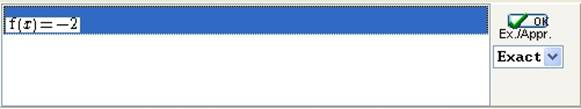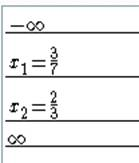﻿ Casyopee - Limit, Derivative, Equation

What they say:   I used Casyopée to help students solve optimisation problems. It allowed me to present students very open tasks. Students explored and found results that they had to prove afterward. Casyopée also helped students reuse basic strategies for solving.
What they say:   Casyopée is faster and more convenient than a calculator.... We have the geometric and algebraic side of the problem at the same time. It is easier to see how a function "reacts." It's useful and interesting.
What they say:   Casyopée makes it easy to calculate a derivative, to factor, to calculate zeros... and have a graph of the function next to it in the same window. It allows on a geometric problem to be able to establish variables that can then be used to study the problem by way of functions...
What they say:   Casyopee is a powerful application that can prove useful to both students and teachers It allows you to use various exploration and modeling tools, with the purpose of studying or teaching mathematical functions.
What they say:   Casyopee comes with lots of features. One of these features is the help provided for proving a function. There is also a feature for writing HTML reports that include the mathematical functions. Casyopee is guaranteed to improve the mathematical knowledge of its users.
What they say:   Besides the concept of number, the concept of function is the most important one in mathematics
What they say:   The notion of function is present in all scientific disciplines, and also in everyday life. Our experience as a teacher shows every day that it is a problem for many students. Situations with Casyopée can also be used outside of a technological environment and everyone will be able to reflect on her professional practice.

In the Algebra tab menu click Create function, and choose domain +  formula. Note that in the entry box, checking existence is now proposed as a default. Enter the formula (x+1)/(3x‑2).Figure 36 - Create function by formula + domain

If you keepas the domain of definition, Casyopée will say after validating the function: “This can lead to errors in the plots or calculations; confirm?”. Edit the domain intoand click.  Casyopée creates a « value of x » x1 here equal to 2/3 and rewrites the domain.
If you created this value before, the Auto button enters the appropriate domain automatically.

 After clicking the Create button the Algebra tab now displays a list of values of x, and a mini-diagram for every function whose existence has been checked.Figure 37 - List of values of x and mini-diagram

Limits

One can obtain values or limits taken by the selected function for the values existing in the x-values list.

Press the function and value at the bottom of the graphic tab.Figure 40 - Symbolic values list

### Derivative

We differentiate our function.Figure 41 - Menu Calculate / derivative

Select by clicking the expression, it appears highlighted. The Calculate button appears. Click this button and choose derivative. A dialogue box asks for confirmation. Select Yes.Figure 42 - Confirmation box for a derivative

Casyopée opens a dialog box offering to confirm or change the domain. It is because the derivative is not always defined on the whole domain of the function. Here we have not to change the domain. Click Evaluate then OK.Figure 43 -Choosing a domain for the derivative

Note Casyopée’s diagnostic about the sign of the new function.Figure 44 - Diagnosing the sign of a function

The derivative appears in the Notepad and in the function list (as a child of the function f). Its formula is displayed in the expression list. The symbolic calculation tools of the Calculate menu allows to obtain equivalent expressions of the derivative.Figure 45 - Derivative

### Solving an equation

In the Algebra tab menu, choose Create equation/ f(x)=k. Enter the equation by choosing  f(x) = in the list and entering -2 in the box, then click Create.A new list displays at the bottom of the Algebra tab. It is the equation list.Figure 47 - Equation list

Click on the equation that you want to have the solutions (it is highlighted). Choose Exact and validate with OK.

The solutions appear in the Notepad and a dialogue box asks if the solutions must be put in the X-values list; click on Yes.Figure 48 - Confirmation box for solutions of an equation       Figure 49 - Solution of an equation in the X-values list

You can easily read the results of the study in the NotePad.

Creation date : 05/10/2014 - 11h04
Category : - help